Q&A - Ask Doubts and Get Answers

Sort by :
Clear All
Q

Q.2.    Find the area of any one door in your house.

suppose the length of door is 2m and breadth is 0.5 m  then the area of door =

Find the area of the floor of your classroom.

If floor length is 3m and breadth is 2m then

Find the perimeter of the following rectangles: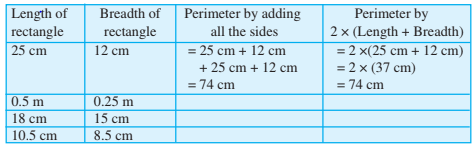Length of rectangle Breadth of rectangle Perimeter by adding all sides perimeter by  25 cm  12 cm =25+12+25+12=74 cm 0.5 cm 0.25cm =0.5+0.25+0.5+0.25=1.5cm 18 cm 15 cm =18+15+18+15=66 cm 10.5 cm 8.5 cm =10.5+8.5+10.5+8.5=38 cm

Find the perimeter of the following figures: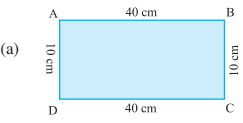Perimeter = AB + BC + CD + DA
= __+__+__+__
= ______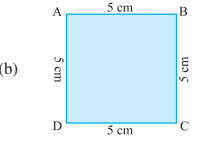Perimeter = AB + BC + CD + DA
= __ + __ + __+ __
= ______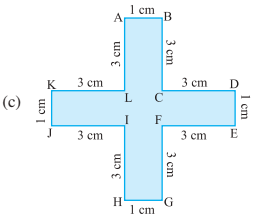Perimeter = AB + BC + CD + DE + EF + FG + GH +HI + IJ + JK + KL + LA
= __ + __ +__ + __ + __ +  __ + __ + __ +__+ __ + __ + __
= ______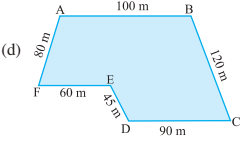Perimeter = AB + BC + CD + DE + EF+ FA
= __ + __ + __ + __ + __ + __
= ______

Find the perimeter of the following figures:                    Perimeter = AB + BC + CD + DA          = 40+10+40+10          = 100                Perimeter = AB + BC + CD + DA       =5+5+5+5=20                  Perimeter = AB + BC + CD + DE + EF + FG + GH +HI + IJ + JK + KL + LA                            =1+3+3+1+3+3+1+3+3+1+3+3=28 cm                       Perimeter = AB + BC + CD +...

Meera went to a park 150 m long and 80 m wide. She took one complete round on its boundary. What is the distance covered by her?

The park is 150m long and 80 m wide. The parks are of the shape of the rectangle. The distance covered by Meera = perimeter of park = 2 (150+80)= 460 m
Exams
Articles
Questions